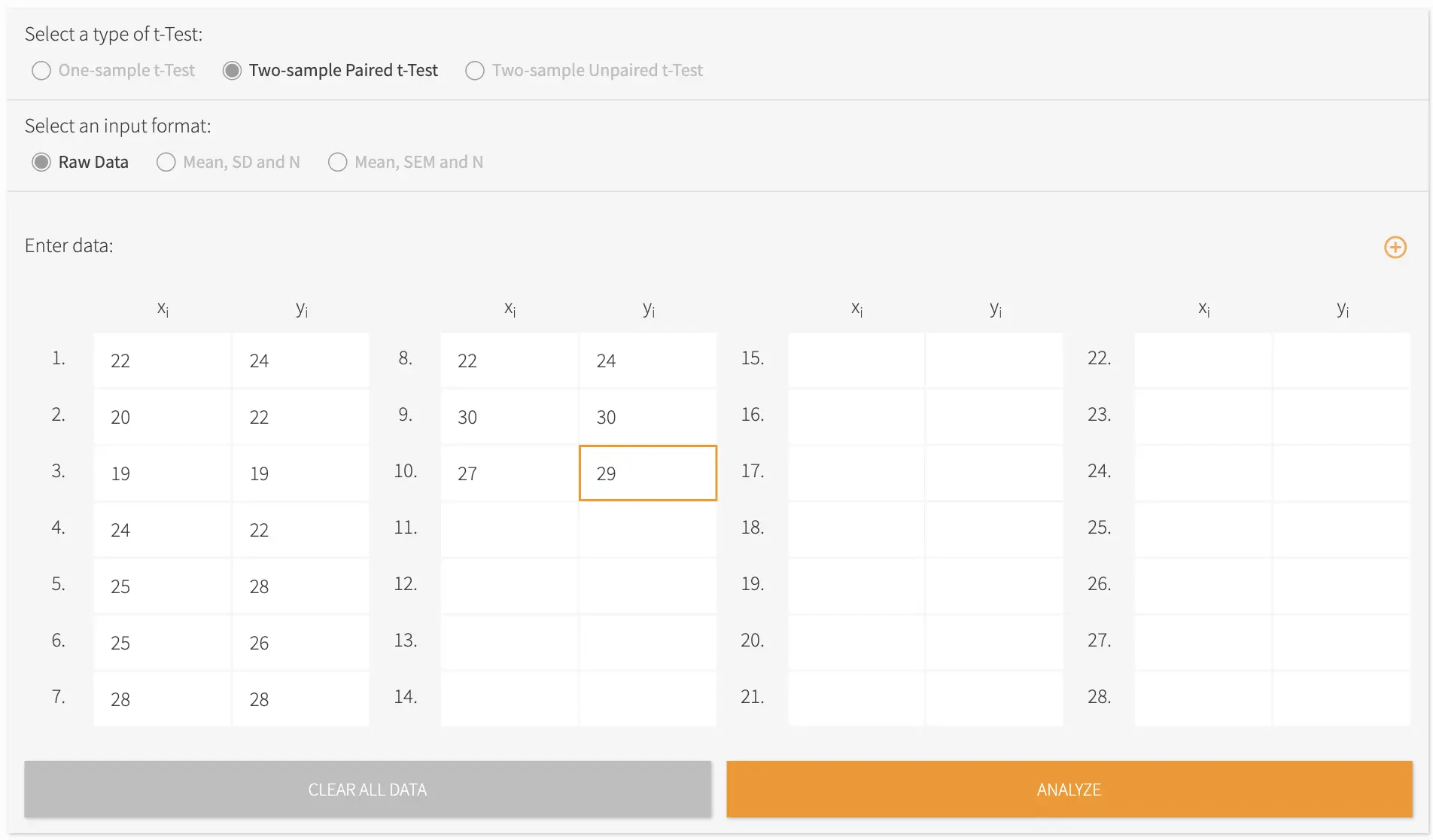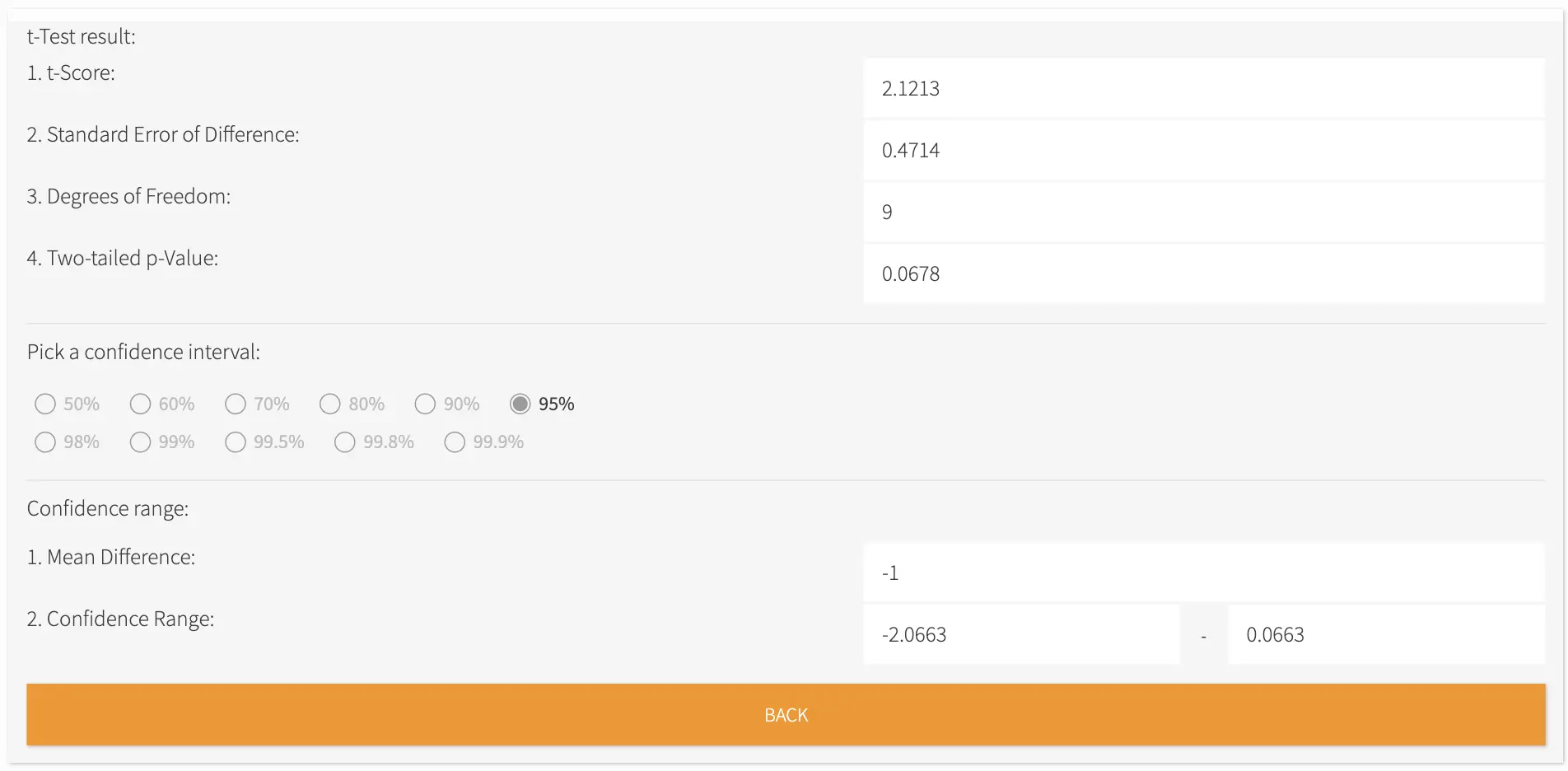# T-test Calculator

• t-test is used to determine, for example, if the means of two data sets differ significantly from each other.
• Our T test calculator is the most sophisticated and comprehensive T-test calculator online.
• Our Student's t-test calculator can do one sample t tests, two sample paired t-tests and two sample unpaired t-tests.
• Results page will give you t-Score, standard error of difference, degrees of freedom, two-tailed p-value, mean difference and confidence range. You can choose the confidence interval.# Best T-Test Calculator Online

-

A t-test is a statistical test most commonly used to determine the difference between the means of two populations. This test can only be applied to one or two groups. Parameters have to be met in order to use a t-test. First, the data points are independent, and second, the data points approximately follow a normal distribution.

One-sample t-tests and two-sample t-tests are the most frequently used type of t-test. One sample t-test is to be used if the mean of a population is equal to some pre-set hypothesized value. On the other hand, the two-sample t-test is used to determine the difference between the means of two independent samples. Thus, this test is also named an unpaired samples t-test, or an independent samples t-test.

There is also the paired t-test, where there are two variables for the same subject which are separated by time. Use paired t-tests to find out the difference between the mean of a population pre and post experimental intervention.

We have our own t-test calculator online that is comprehensive but very easy to use. Using our t-test calculator, you can do one-sample t-tests, two-sample paired t-tests, and two-sample unpaired t-tests. All you have to do is select the type of test, select an input format, and enter the values.Figure 1. Sample paired t-test dataFigure 2. Sample t-test output

#### T-test calculator worksheets & handouts

We’ve listed a few t-test calculator worksheets that you can try out for free. Have fun solving!